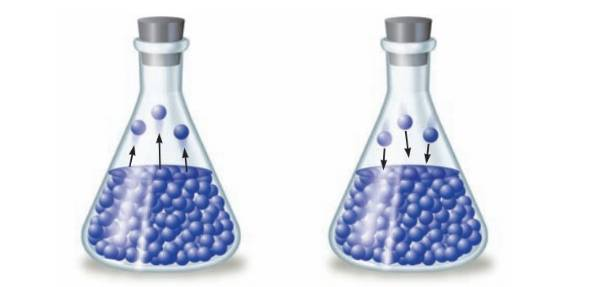Chapter 17, Problem 13ALQ### Introductory Chemistry: A Foundati...

9th Edition
Steven S. Zumdahl + 1 other
ISBN: 9781337399425

#### Solutions

Chapter
Section### Introductory Chemistry: A Foundati...

9th Edition
Steven S. Zumdahl + 1 other
ISBN: 9781337399425
Textbook Problem
1 views

# . Explain why the development of a vapour pressure above a liquid in a closed container represents an equilibrium. What are the opposing processes? How do we recognize when the system has reached a state of equilibrium?Interpretation Introduction

Interpretation:

The reason as to why the development of a vapor pressure above a liquid in a closed container represents an equilibrium is to be explained. The opposing processes are to be defined. The stage at which state of equilibrium for the system has reached is to be recognized.

Concept Introduction:

The vapor pressure is the pressure exerted by particles in vapor phase above the liquid surface. The equilibrium between liquid phase and vapor phase is represented as,

AlAg.

Explanation

When a liquid is kept in a closed container, initially the particles in liquid phase gain some thermal energy and start transferring from liquid phase to vapor phase until a certain point reached. After that, the particles present in liquid phase starts converting back into liquid state. The rate at which particles are transferring from liquid phase to vapor phase is equal to the rate at which particles are transferring from vapor phase to liquid phase, therefore, the development of the vapor pressure above the liquid in the closed container represents equilibrium...

### Still sussing out bartleby?

Check out a sample textbook solution.

See a sample solution

#### The Solution to Your Study Problems

Bartleby provides explanations to thousands of textbook problems written by our experts, many with advanced degrees!

Get Started